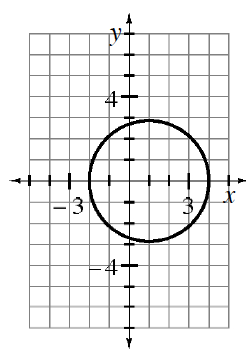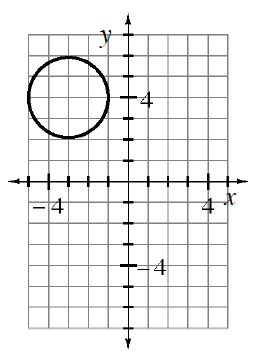### Home > CCA2 > Chapter 6 > Lesson 6.2.3 > Problem6-134

6-134.

Write the equation of each circle graphed below.

Recall the general equation of a circle: $(x−h)^2+(y−k)^2=r^2$ where $(h,k)$ is the center of the circle and $r$ is the radius.

1.Write in graphing form:
$(x−1)^2+y^2=9$

2.